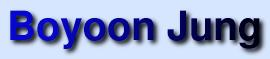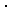Boyoon Jung's Library Manual

# bj::OccupancyMap Class Reference [Robot Programming]

A local occupancy grid map. More...

`#include <occupancy_map.h>`

List of all members.

## Public Member Functions

OccupancyMap (double map_size=5.0, double grid_size=0.1, double move_step=1.0, double cx=0.0, double cy=0.0)
A constructor.
~OccupancyMap (void)
A destructor.
void move (double x, double y, double a)
Move the local coordinates.
void update (double distance, int increase=2, int decrease=1, double dx=0.0, double dy=0.0, double da=0.0)
Update the map based on distance sensor.
void update (double x, double y, double a, double distance, int increase=2, int decrease=1, double dx=0.0, double dy=0.0, double da=0.0)
Move the local coordinates, and update the map.
void reset (double cx=0.0, double cy=0.0)
Reset the local map.
bool copy (double **data, double radius)
Copy a specific region of the grid map.
bool read (int file)
Load a map from a file.
bool write (int file)
Dump the current map into a file.
double value (int i, int j)
double mapSize (void)
double gridSize (void)
double moveStep (void)
double centerX (void)
double centerY (void)
double x (void)
double y (void)
double heading (void)

## Protected Member Functions

void increase (int x, int y, int step=1)
Increase the certainty of a cell.
void decrease (int x, int y, int step=1)
Decrease the certainty of a cell.
bool read_nbytes (int file, unsigned char *buffer, size_t length)
Read N bytes from a file.

## Detailed Description

A local occupancy grid map.

OccupancyMap class implements a local occupancy grid map. The range of certainty values are from 0 (for open/unknown) to 255 (for occupied).

## Constructor & Destructor Documentation

 bj::OccupancyMap::OccupancyMap ( double map_size = `5.0`, double grid_size = `0.1`, double move_step = `1.0`, double cx = `0.0`, double cy = `0.0` )

A constructor.

Parameters:
 map_size The size of a local map [in meters]. grid_size The size of a single grid [in meters]. move_step The step size for scrolling a map [in meters]. cx The initial position of a robot in the local map [in meters]. cy The initial position of a robot in the local map [in meters].

 bj::OccupancyMap::~OccupancyMap ( void )
 A destructor.

## Member Function Documentation

 double bj::OccupancyMap::centerX ( void ) ` [inline]`

 double bj::OccupancyMap::centerY ( void ) ` [inline]`

 bool bj::OccupancyMap::copy ( double ** data, double radius )
 Copy a specific region of the grid map.

 void bj::OccupancyMap::decrease ( int x, int y, int step = `1` ) ` [inline, protected]`
 Decrease the certainty of a cell.

 double bj::OccupancyMap::gridSize ( void ) ` [inline]`

 double bj::OccupancyMap::heading ( void ) ` [inline]`

 void bj::OccupancyMap::increase ( int x, int y, int step = `1` ) ` [inline, protected]`
 Increase the certainty of a cell.

 double bj::OccupancyMap::mapSize ( void ) ` [inline]`

 void bj::OccupancyMap::move ( double x, double y, double a )
 Move the local coordinates.

 double bj::OccupancyMap::moveStep ( void ) ` [inline]`

 bool bj::OccupancyMap::read ( int file )
 Load a map from a file.

 bool bj::OccupancyMap::read_nbytes ( int file, unsigned char * buffer, size_t length ) ` [inline, protected]`
 Read N bytes from a file.

 void bj::OccupancyMap::reset ( double cx = `0.0`, double cy = `0.0` )
 Reset the local map.

 void bj::OccupancyMap::update ( double x, double y, double a, double distance, int increase = `2`, int decrease = `1`, double dx = `0.0`, double dy = `0.0`, double da = `0.0` ) ` [inline]`
 Move the local coordinates, and update the map.

 void bj::OccupancyMap::update ( double distance, int increase = `2`, int decrease = `1`, double dx = `0.0`, double dy = `0.0`, double da = `0.0` )
 Update the map based on distance sensor.

 double bj::OccupancyMap::value ( int i, int j ) ` [inline]`

 bool bj::OccupancyMap::write ( int file )
 Dump the current map into a file.

 double bj::OccupancyMap::x ( void ) ` [inline]`

 double bj::OccupancyMap::y ( void ) ` [inline]`

The documentation for this class was generated from the following file:
ResearchProjectsPublications

ToolsBjLib ManualPlayer/StagePyPlayerMicroStrain IMUGPS/DGPSParticle Filter

PersonalWelcomeCurriculum VitaeResumeContact

Outside LinksUSCUSC CS DeptCRESRobotics LabRESL